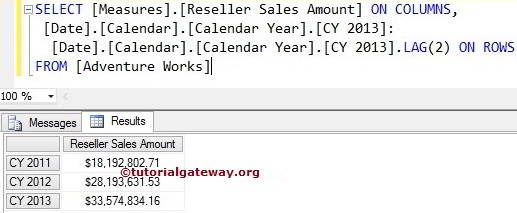# MDX PrevMember Function

The MDX PrevMember function will return the same level member at the Previous position.

## MDX Prevmember Function Syntax

The basic syntax of the Prevmember Function in Multidimensional Expression is as shown below:

`Member_Expression.PREVMEMBER`

Member_Expression: Any Multidimensional Expression that returns valid Member.

The MDX Prevmember Function is used to move backward between Members at the same level. For example, If we mentioned City member as Member_Expression, the Prevmember function will move backward between remaining Cities. It will not go Level Up (State – Province) or Level down (Postal Code).

For this MDX Prevmember function example, we are going to use below shown data.

## MDX Prevmember Function Example

In this example, we will show you, How to write the PrevMember function in a Multidimensional Expression query. The following query will return the Reseller Sales amount of the Calendar Year 2012 because [CY 2013].PrevMember will look for a year before 2013, which is 2012.

```SELECT [Date].[Calendar].[Calendar Year].[CY 2013].PREVMEMBER ON COLUMNS,
[Measures].[Reseller Sales Amount] ON ROWS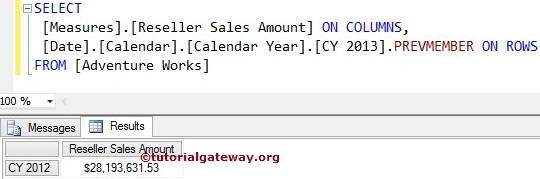## MDX Previous member Function Example 2

In this example, we will show you how to write the multiple PrevMember functions in a single Multidimensional Expression query. The following MDX query will return the Reseller Sales amount of the Calendar Year 2011.

```SELECT [Date].[Calendar].[Calendar Year].[CY 2013].PREVMEMBER.PREVMEMBER ON 0
,[Measures].[Reseller Sales Amount] ON ROWS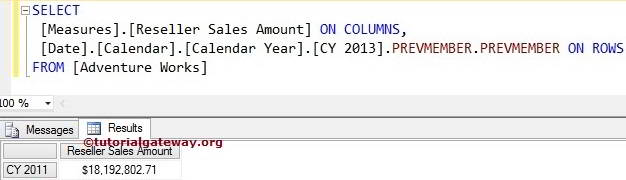First, [CY 2013].PrevMember will look for the year before 2013, which is 2012

`[Date].[Calendar].[Calendar Year].[CY 2013].PREVMEMBER`

Second, [CY 2011].PrevMember.PrevMember will look for the year before 2012, which is 2011

`[Date].[Calendar].[Calendar Year].[CY 2013].PREVMEMBER.PREVMEMBER`

## Finding Range Using MDX Prevmember Function

How to find the Range using Prevmember function?. The following query will return the Reseller Sales amount from Calendar Year 2011 to 2013 because we used the range symbol (:) between [CY 2013] and [CY 2013].PrevMember.PrevMember

```SELECT
[Date].[Calendar].[Calendar Year].[CY 2013]:
[Date].[Calendar].[Calendar Year].[CY 2013].PREVMEMBER.PREVMEMBER ON 0
,[Measures].[Reseller Sales Amount] ON ROWS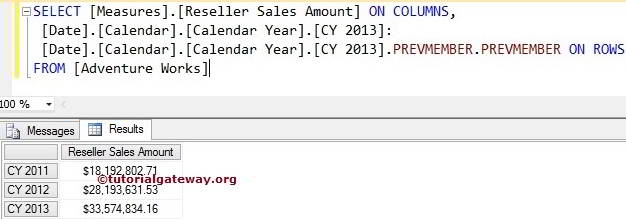## MDX Prevmember Function Alternative 1

In this example, we are going to use Lead Function with Negative integer value. Please refer MDX Lead Function article to understand the function

```SELECT
[Date].[Calendar].[Calendar Year].[CY 2013]:
,[Measures].[Reseller Sales Amount] ON ROWS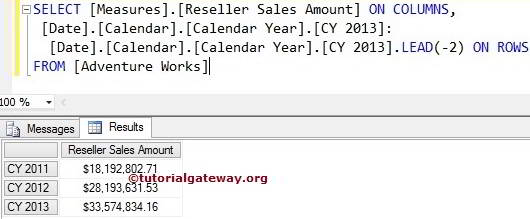## Prevmember Function Alternative 2

In this example, we are going to use Lag Function with Positive integer value. Please refer MDX Lag Function article to understand the function

```SELECT
[Date].[Calendar].[Calendar Year].[CY 2013]:
[Date].[Calendar].[Calendar Year].[CY 2013].LAG(2) ON 0
,[Measures].[Reseller Sales Amount] ON ROWS